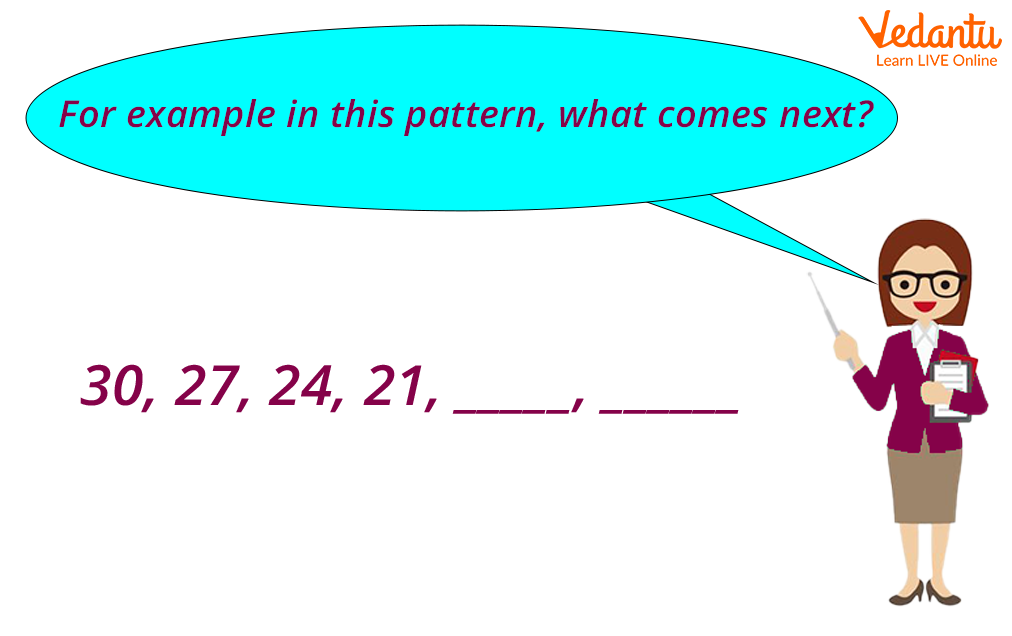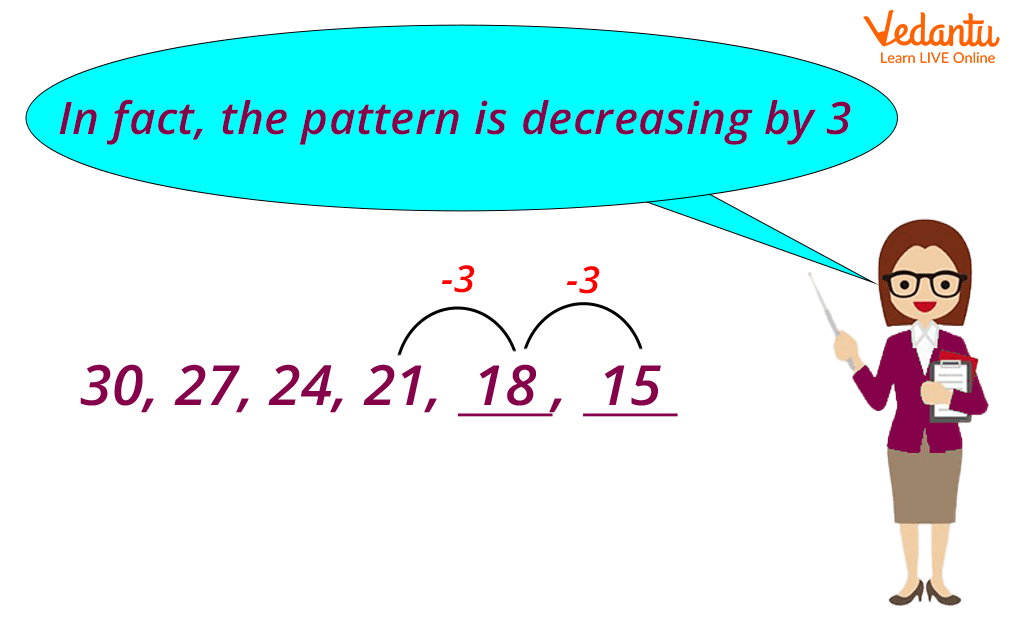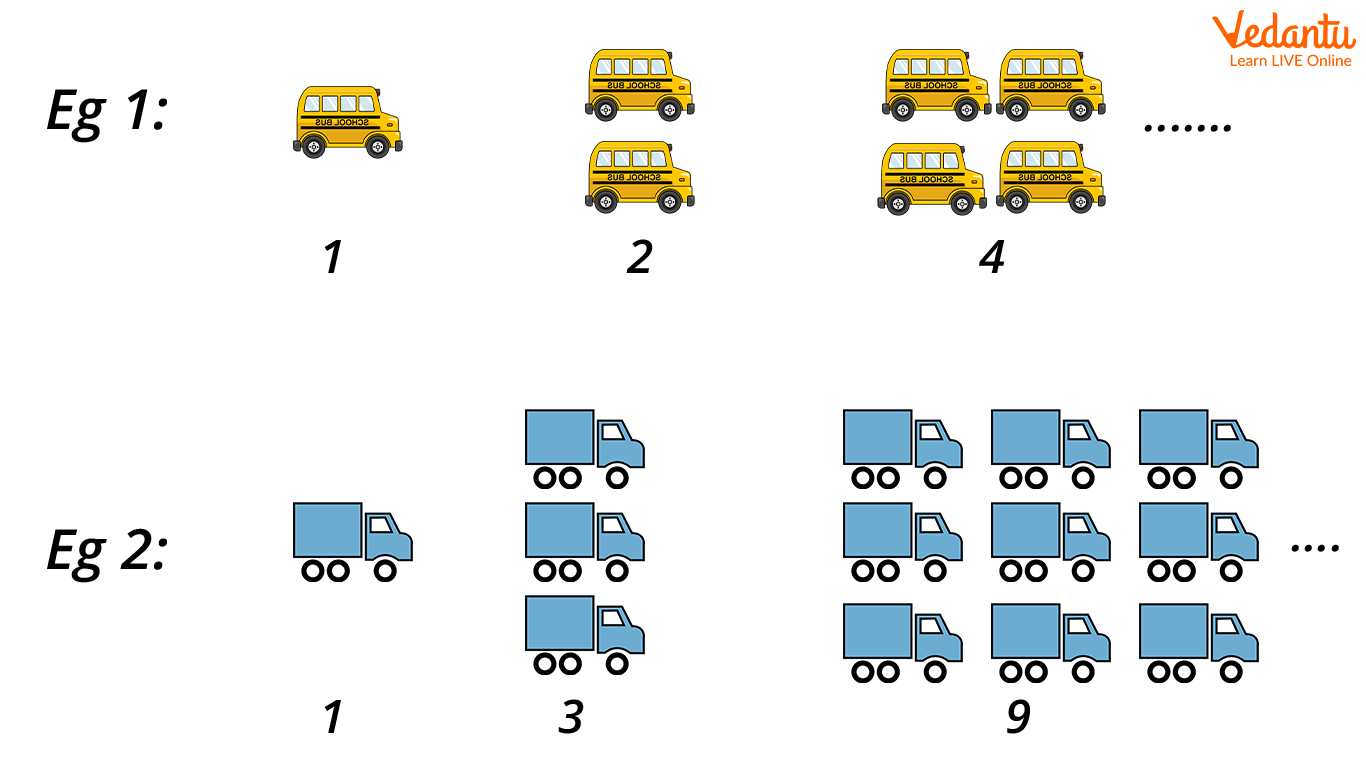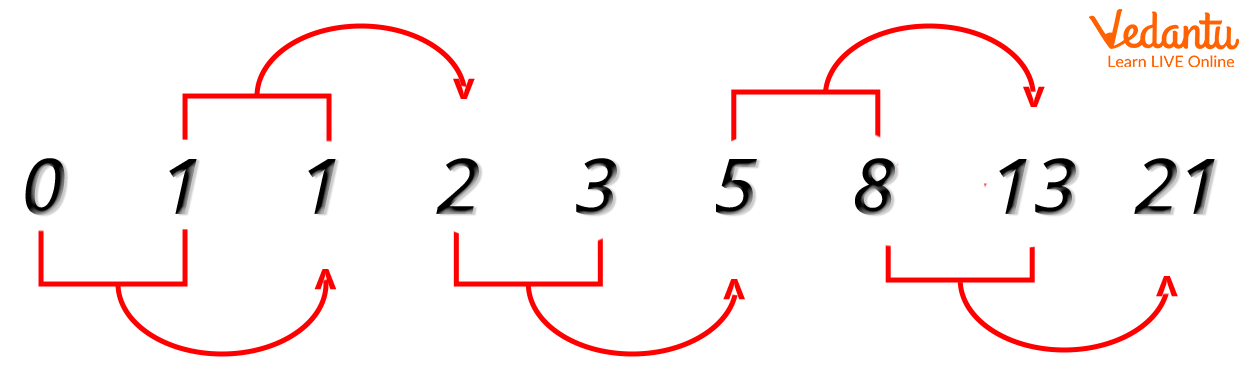Courses
Courses for Kids
Free study material
Offline Centres
More

# What is a Sequence?Last updated date: 30th Nov 2023
Total views: 150.9k
Views today: 4.50k## Sequence: Observing the Logic in Every Pattern

Do you know how to predict which number comes next in a series of numbers? Well, patterns are all around us. Patterns can be repeated items, shapes, colours, images, or numbers. Sometimes a set of things is repeated to create a pattern. Number patterns are fun because you need to look carefully for clues to complete them. If you are intrigued and want to know what number sequence is or how you can identify a pattern, continue reading through the content below.

So, what is the number sequence? Let’s understand with the help of simple activities given below.

## Activity

Here is a number pattern, or a sequence of numbers. Find out what comes next?

1, 2, 3, 4, 5, __

This is a fairly easy pattern because we count this one all the time. It’s 6.

The number 6 completes this pattern.

Here is another one.

Let’s find the next number by following the pattern 2, 4, 6, 8, ___.

We have 2, 4, 6, 8. If you look for clues, we see that each number in this sequence exceeds its previous number by 2. That’s the clue.

⇒ 2 + 2 is 4.

⇒ 4 + 2 is 6.

⇒ 6 + 2 is 8.

We should add 2 to 8 to give us the next number. Therefore, the next number is 10.

Number patterns can also work in reverse.Number Pattern

When you look closely, you will see that the numbers are decreasing. It means that in this pattern, the numbers are not adding on. It's subtracting.Number Pattern

30 − 3 is 27.

27 − 3 is 24.

24 − 3 is 21.

21 − 3 is 18.

81 − 3 is 15.

## Things to Remember

• Number pattern: These are the numbers that follow a particular sequence or pattern.

• Growing pattern: If the numbers are getting bigger, then it is a growing pattern.

• Shrinking pattern: If the numbers are getting smaller, then it will be a shrinking pattern.

• Repeating pattern: When the same number grouping is observed over and over again.

## Types of Sequences

There are different types of sequences based on the pattern among the terms. The following are the most familiar sequences.

1. Arithmetic Sequence:

In an arithmetic sequence, the common difference between any two successive terms remains the same. Note the common difference can be either negative or positive.

For example: 5, 10, 15, 20, 25, 30, ...

In this example, the common difference between any two successive terms is 5.Arithmetic Sequence

2. Geometric Sequence:

A geometric sequence is one in which the common ratio of any two successive terms is the same. Multiplying the first term with the common ratio gives the second term and multiplying the second term with the common ratio gives the third term and so on.

For example: 2, 4, 8, 16, 32, 64…

Here, the common ratio between any two successive terms is 2.Geometric Sequence

3. Harmonic Sequence:

A sequence is said to be a harmonic sequence if the reciprocals of the terms are in an arithmetic sequence.

For example: $\frac{1}{5}, \frac{1}{10}, \frac{1}{15}, \frac{1}{20}, \frac{1}{25}, \frac{1}{30},....$

If you take the reciprocals of each term in this sequence, we get 5, 10, 15, 20, 25, 30, … which are in an arithmetic sequence.

4. Fibonacci Sequence:

Fibonacci numbers are a fascinating number series in which each element is created by adding two preceding elements, with the sequence beginning with 0 and 1. The first term is 0 and the second term is 1. The third term will be obtained by adding the first and second term which is equal to 0+1 = 1. The fourth term will be equal to the sum of the third and second terms, i.e., 1 + 1 = 2, and so on.Fibonacci Sequence

## Conclusion

Number sequencing is a process in a child's development of number sense. This is because it involves calculating, thinking, and reasoning. The steps, however, can be confusing to children. So, rather than forcing children to take a compulsive learning course, we can involve them in pleasant games and activities to make learning easier. Children can master arithmetic reasoning if they are familiar with numerical sequencing. To teach your young ones similar Maths topics in a fun way and help create a strong mathematical base, visit our website now.

## FAQs on What is a Sequence?

1. What is the definition of sequence?

A series is a collection of numbers that are arranged in a specific order. Each number in a sequence is referred to as a term. The position of each term in a sequence is numbered in order like the first term, second term, third term, and so on.

Example of sequence: 50,100, 150, 200, 250 ...

2. What is a finite and an infinite sequence?

Finite sequence: If a sequence has a finite number of terms, it is called a finite sequence. In any finite sequence, the first term and the last term are defined.

For example, 4, 8, 12, 16,...,64 is a finite sequence. We can see 4 is the first term and 64 is the last term of this finite sequence.

Infinite sequence: If a sequence has an infinite number of terms, it is called an infinite sequence. In any infinite sequence, there is no particular last term.

For example, 4, 8, 12, 16,........is an infinite sequence. Here, 4 is the first term. The dots at the end represent the sequence will continue infinitely and there will be no last term.

3. What are increasing and decreasing sequences?

Increasing sequence: An increasing sequence is a sequence in which each term is greater than the previous term.

For example, 3, 6, 9, 12, ….., 24.

Decreasing sequence: A decreasing sequence is a sequence in which each term is lesser than the previous term.

For example, 10, 9, 8, 7, 6, ….., -10.